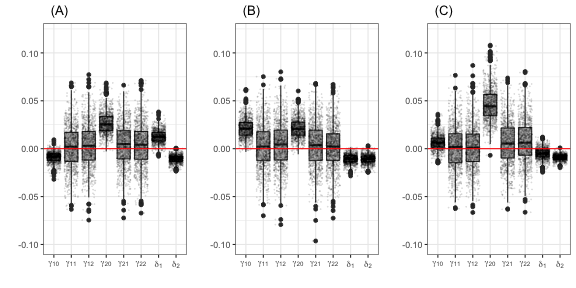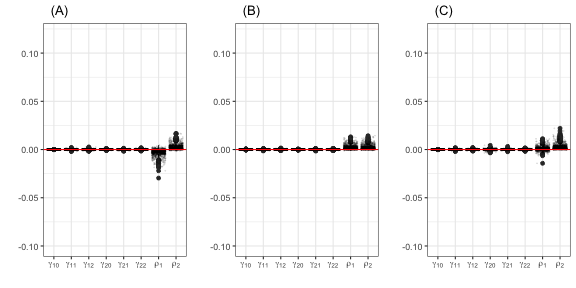# 1 A short Monte Carlo exercise: spsur vs spse.

The goal of this vignette is to present the results obtained in a Monte Carlo exercise to evaluate the performance of the Maximum Likelihood (ML) estimation of three spatial SUR models using the R-package spsur . The results will be compared with the same estimation using the spse R-package when it is possible. We compare the two basic spatial SUR models, named SUR-SLM and SUR-SEM. In the case of SUR-SARAR, we only present the results obtained with spsur because the estimation of this model is not available with spse.

The design of the Monte Carlo is as follows: We simulate a spatial SUR model with two equations (G = 2), where each equation includes an intercept and two explanatory variables plus the corresponding spatial terms. For the general model the equation is:

$\begin{equation} y_i = (I_N-\rho_iW)^{-1}(\beta_{i0} + X_{i1}\beta_{i1} + X_{i2}\beta_{i2} + (I_N-\lambda_iW)^{-1}\epsilon_i); \ cov(\epsilon_i,\epsilon_j)=\sigma_{ij} ; \ i=1,2 (\#eq:sur) \end{equation}$

During the experiment, the $$\beta$$ parameters are fixed for every model taking the values $$\beta_{10}=\beta_{20}=1$$; $$\beta_{11}=\beta_{21}=2$$ and $$\beta_{12}=\beta_{22}=3$$. The variance-covariance matrix $$\Sigma=(\sigma_{ij})$$ is defined by $$\sigma_{ij}=0.5 \ (i \neq j)$$ and $$\sigma_{ii}=1 \ (i=1,2)$$. Two sample sizes, small and medium, are choosen (N=52, 516). A regular hexagonal layout is selected, from which the W matrix is obtained, based on the border contiguity between the hexagons (rook neighborhood type). Figure $$\ref{Fig:geometry}$$ shows the hexagonal lattices for the case of N = 516. The $$X_{ij}$$ (i,j=1,2) variables are drawn from an independent U(0,1), and the error terms from a bivariate normal distribution with a variance-covariance matrix $$\Sigma$$. For all the experiments, 1,000 replications are performed.

Several combinations of parameters are selected to evaluate the performance of the ML algorithm under different levels of spatial dependence.

SUR-SLM: $$(\rho_1,\rho_2)=(-0.4,0.6);(0.5,0.5);(0.2,0.8)$$ and $$(\lambda_1,\lambda_2)=(0,0)$$

SUR-SEM: $$(\rho_1,\rho_2)=(0,0)$$ and $$(\lambda_1,\lambda_2)=(-0.4,0.6);(0.5,0.5);(0.2,0.8)$$

SUR-SARAR: $$(\rho_1,\rho_2)=(\lambda_1,\lambda_2)=(-0.4,0.6);(0.5,0.5);(0.2,0.8)$$

These spatial processes have been generated using the function dgp_spsur(), available in the spsur package. To evaluate the performance of the Maximum Likelihood estimation, we report bias and root mean-squared errors (RMSE) for all the combinations of the spatial parameters.

If spsur and spse needed to be installed, the first one is available in the CRAN repository and the second one can be installed from the following GitHub repository:

# install_github("gpiras/spse",force = TRUE)
library(spse)

The package sf is used to generate hexagonal and regular lattices with the number of hexagons prefixed and spdep to obtain the W matrix based on a common border.

library(sf)
library(spdep)
sfc <- st_sfc(st_polygon(list(rbind(c(0,0),c(1,0),c(1,1),c(0,1),c(0,0)))))
hexs.N52.sf <- st_sf(st_make_grid(sfc, cellsize = .19, square = FALSE))
hexs.N525.sf <- st_sf(st_make_grid(sfc, cellsize = .05, square = FALSE))
listw.N52 <- as(hexs.N52.sf, "Spatial") %>%
poly2nb(queen = FALSE) %>% nb2listw()
listw.N525 <- as(hexs.N525.sf, "Spatial") %>%
poly2nb(queen = FALSE) %>% nb2listw()

# 2 Maximum Likelihood estimation of SUR-SLM models

This section presents the results of a Monte Carlo exercise for the ML estimation of SUR-SLM models.

$\begin{equation} y_i = (I_N-\rho_iW)^{-1}(\beta_{i0} + X_{i1}\beta_{i1} + X_{i2}\beta_{i2} + \epsilon_i) \ ; \ cov(\epsilon_i,\epsilon_j)=\sigma_{ij} \ ; \ \ i=1,2 (\#eq:sur-sem) \end{equation}$

Table 1 shows the mean of the bias and the RMSE of the $$\beta's$$ and $$\rho's$$ parameters for the 1,000 replications. In general, all the results are coherent. The estimations with both R-packages show similar results. The highest bias is observed in the estimates of the intercept of the second equation for both packages. When the model is estimated with spsur the maximum bias is reached for N = 52 and when the model is estimated with spse the maximum bias corresponds to N = 516. In general, the results confirm that for both packages, the estimates of the parameters of spatial dependence present low biases. The RMSE values decrease when the sample size increases, as expected.

Table 1: SUR-SLM Bias and RMSE. Maximum Likelihood
Pack. N $$\rho_1$$ $$\rho_2$$ $$\hat\beta_{10}$$ $$\hat\beta_{11}$$ $$\hat\beta_{12}$$ $$\hat\beta_{20}$$ $$\hat\beta_{21}$$ $$\hat\beta_{22}$$ $$\hat\rho_1$$ $$\hat\rho_2$$
spsur 52 -0.4 0.6 0.009 0.001 0.001 0.036 0.001 -0.004 -0.007 -0.012
-0.4 0.6 (0.156) (0.126) (0.126) (0.208) (0.133) (0.132) (0.077) (0.054)
0.5 0.5 0.027 -0.002 0.001 0.027 0.001 0.000 -0.012 -0.011
0.5 0.5 (0.201) (0.129) (0.129) (0.199) (0.132) (0.126) (0.061) (0.059)
0.2 0.8 0.017 -0.006 -0.001 0.065 0.006 0.002 -0.011 -0.012
0.2 0.8 (0.178) (0.124) (0.126) (0.276) (0.129) (0.126) (0.071) (0.040)
516 -0.4 0.6 -0.002 0.000 -0.000 0.001 0.002 0.001 -0.002 -0.001
-0.4 0.6 (0.047) (0.038) (0.040) (0.058) (0.040) (0.041) (0.025) (0.015)
0.5 0.5 0.000 -0.001 0.001 -0.001 -0.001 -0.001 -0.001 -0.001
0.5 0.5 (0.057) (0.039) (0.041) (0.058) (0.039) (0.041) (0.017) (0.017)
0.2 0.8 0.003 0.001 -0.000 0.007 0.001 0.000 -0.001 -0.001
0.2 0.8 (0.052) (0.038) (0.039) (0.068) (0.038) (0.040) (0.022) (0.010)
spse 52 -0.4 0.6 0.018 -0.001 -0.002 0.007 -0.004 -0.009 -0.021 -0.001
-0.4 0.6 (0.159) (0.143) (0.143) (0.205) (0.147) (0.144) (0.083) (0.054)
0.5 0.5 0.002 -0.003 -0.003 0.005 -0.003 -0.005 0.000 0.000
0.5 0.5 (0.201) (0.149) (0.146) (0.200) (0.150) (0.141) (0.063) (0.061)
0.2 0.8 0.011 -0.002 -0.004 0.008 -0.001 -0.009 -0.006 -0.001
0.2 0.8 (0.180) (0.143) (0.149) (0.267) (0.149) (0.153) (0.074) (0.039)
516 -0.4 0.6 0.007 -0.001 -0.004 -0.025 -0.002 -0.003 -0.014 0.009
-0.4 0.6 (0.049) (0.044) (0.045) (0.063) (0.046) (0.048) (0.030) (0.018)
0.5 0.5 -0.021 -0.003 -0.003 -0.022 -0.004 -0.003 0.010 0.010
0.5 0.5 (0.061) (0.045) (0.047) (0.063) (0.045) (0.046) (0.020) (0.020)
0.2 0.8 -0.004 0.000 -0.001 -0.039 -0.005 -0.007 0.004 0.008
0.2 0.8 (0.053) (0.044) (0.044) (0.078) (0.043) (0.045) (0.023) (0.013)

Figure 1 shows the boxplots of $$\gamma_{ij}=\hat\beta_{ij}^{spsur}-\hat\beta_{ij}^{spse}$$ and $$\delta_i=\hat\rho_{i}^{spsur}-\hat\rho_{i}^{spse}$$, the difference between estimated parameters ‘model to model’ for N = 516 (the superscript indicates the package used to estimate the coefficient). These boxplots confirm that the main differences are founded in the intercept of the second equation.Figure 1: Difference between parameters ‘model to model’ SUR-SLM (N=516). (A) $$\rho_1=-0.4; \rho_2=0.6$$ ; (B) $$\rho_1=0.5; \rho_2=0.5$$ ; (C) $$\rho_1 = 0.2; \rho_2 = 0.8$$

# 3 Maximum Likelihood estimation of SUR-SEM models

Table 1 shows the results of the bias and RMSE for the estimation of an SUR-SEM model with both R-packages. In general terms, the biases of the estimated parameters are lower than 0.01 in absolute values for all $$\beta$$ parameters. The estimation of the $$\lambda's$$ parameters for small sample (N = 52) has a bias higher than 0.01 with a tendency toward the underestimation in all the cases. For medium sample sizes (N = 516), the bias is lower than 0.01. The RMSE decreases when the sample size increase as expected.

As in the case of SUR-SLM, the Figure 2 shows the difference between the parameters estimated with spsur and spse for N = 516. These boxplots show that the biases in the SUR-SEM are lower than in the SUR-SLM for all the parameters.Figure 2: Difference between parameters ‘model to model’ SUR-SEM N=516. (A) $$\lambda_1=-0.4; \lambda_2=0.6$$ ; (B) $$\lambda_1=0.5; \lambda_2=0.5$$ ; (C) $$\lambda_1 = 0.2; \lambda_2 = 0.8$$

# 4 Maximum Likelihood estimation of SUR-SARAR models

Table 3 shows the results obtained for the bias and RMSE for the LM estimation of SUR-SARAR models. For this model, only the results obtained with the spsur package can be shown because this specification is not available for the spse package. As in the case of the estimations of the SUR-SLM and SUR-SEM models the worst results in terms of bias and RMSE are obtained when the sample size is small (N = 52). In the case of N = 52 the $$\lambda's$$ parameters are underestimated. This underestimation disappears when the sample size is medium (N = 516).

Table 3: SUR-SARAR Bias and RMSE (in brackets). Maximum Likelihood with spsur
N $$\rho_1;\lambda_1$$ $$\rho_2;\lambda_2$$ $$\hat\beta_{10}$$ $$\hat\beta_{11}$$ $$\hat\beta_{12}$$ $$\hat\beta_{20}$$ $$\hat\beta_{21}$$ $$\hat\beta_{22}$$ $$\hat\rho_1$$ $$\hat\rho_2$$ $$\hat\lambda_1$$ $$\hat\lambda_2$$
52 -0.4 0.6 0.005 0.003 0.002 0.032 -0.002 -0.012 -0.003 -0.009 -0.077 -0.104
52 -0.4 0.6 (0.121) (0.127) (0.124) (0.439) (0.133) (0.131) (0.080) (0.083) (0.252) (0.210)
52 0.5 0.5 0.027 -0.007 -0.005 0.014 -0.003 -0.004 -0.010 -0.003 -0.094 -0.117
52 0.5 0.5 (0.365) (0.130) (0.129) (0.345) (0.130) (0.128) (0.089) (0.084) (0.229) (0.231)
52 0.2 0.8 0.010 -0.007 -0.004 0.098 -0.002 -0.009 -0.006 -0.013 -0.103 -0.093
52 0.2 0.8 (0.214) (0.126) (0.127) (0.931) (0.122) (0.123) (0.083) (0.080) (0.253) (0.177)
516 -0.4 0.6 -0.001 0.001 -0.000 -0.002 0.002 0.000 -0.001 -0.001 -0.008 -0.010
516 -0.4 0.6 (0.036) (0.037) (0.039) (0.127) (0.039) (0.040) (0.025) (0.026) (0.076) (0.053)
516 0.5 0.5 -0.002 -0.001 0.000 -0.006 -0.001 -0.002 -0.001 -0.001 -0.008 -0.012
516 0.5 0.5 (0.106) (0.038) (0.041) (0.106) (0.038) (0.041) (0.026) (0.026) (0.058) (0.059)
516 0.2 0.8 0.002 0.001 -0.000 0.021 -0.000 -0.001 -0.000 -0.001 -0.013 -0.009
516 0.2 0.8 (0.064) (0.038) (0.038) (0.262) (0.036) (0.038) (0.025) (0.025) (0.070) (0.041)

# 5 Conclusion

This vignette shows the results of a sort Monte Carlo exercise to evaluate the ML estimation of three spatial SUR models, SUR-SLM, SUR-SEM, and SUR-SARAR. The first two models are estimated with the spsur and spse packages and the results are compared. In the case of the SUR-SARAR model only the results using the spsur are presented because the estimation of SUR-SARAR is no available.

In general, both packages present admissible results. When comparing the estimates of the coefficients for SUR-SLM some differences emerge, mainly in the estimation of the intercepts. In the case of SUR-SEM both R-packages give similar results for small and medium sample sizes.

A full Monte Carlo using irregular lattices, alternative W matrices, and non-ideal conditions would shed more light on the performance of the ML algorithm implemented in both R-packages.